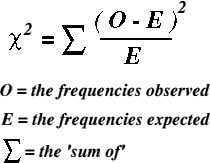# Category Archives: Statistical Methods (1b)

## Statistical Methods – McNemar’s Test

What is McNemar’s Test?

The McNemar Chi-Square test is used in situations where data is not independent.

Specifically data is either matched (e.g. 2 people matched on similar characteristics, typically have different exposure – outcomes compared) or before-after data (e.g. one person measured before and after exposure – outcomes compared)

• It is a non-parametric test
• Tests the difference between paired proportions
• Uses RAW numbers

Formulae… How to calculate McNemar’s test

1.Data should be placed into a trusty 2×2 table
2. As this is paired data, we will be ignoring concordant cells (a and d)
3. Plug b + c numbers into the formulae above… et voila!

As with the Chi-Square test, the cut-off point for 95% significance level is 3.85 (which is 1.96 squared).  Therefore if the result is bigger than 3.85, we can say it is statistically significant at the 95% level (where p=0.05)

Here is a handy flow-chart to help decided which test to use.…This was ‘borrowed’ from this useful website on commonly used statistical tests

## Statistical Methods – Chi-Square and 2×2 tables

Definition

When data is binary (i.e. exposure and outcome have only 2 options) the data can be plotted into a trusty 2×2 table (*they really are trusty – they pop up all over the place!)

The Chi-Square statistic (denoted x^2) is a non-parametric test which examines whether there is an association between 2 variables of a sample.  It determined if a distribution of observed frequencies differ from expected frequencies

• Measured variables much be independent
• Values of independent and dependent variables must be mutually exclusive
• Data must be raw numbers (e.g. nominal or ordinal)
• Data must be randomly drawn from the population
• Observed frequencies must not be too small (as expected No. must be >5… if <5 then Fisher’s Exact Test must be used)

Formulae, and how to…1. For each observed number, calculate the expected number = [(row total x col total) / table total]
2. Subtract expected from observed [O-E]
3. Square the result and divide by the expected number [(O-E)^2 / e]
4. Chi-Square = Sum of results for all cells

In 2×2 table the cut-off for 95% significance level (p = 0.05) = 3.84 (1.96^2).

If Chi-square is less than 3.84 we can say the result was statistically significant (at the 95% level)

The bigger the chi-square result, the more statisically significant it will be

DONE!

## Statistical Methods – Standard Error and Confidence Intervals

This post covers the 3 applications of standard error required for the MFPH Part A; mean, proportions and differences between proportions (and their corresponding confidence intervals)…

a) What is the etandard error (SE) of a mean?

The SE measures the amount of variability in the sample mean.  It indicated how closely the population mean is likely to be estimated by the sample mean.

(NB: this is different from Standard Deviation (SD) which measures the amount of variability in the population.  SE incorporates SD to assess the difference beetween sample and population measurements due to sampling variation)

• Calculation of SE for mean = SD / sqrt(n)

…so the sample mean and its SE provide a range of likely values for the true population mean.

How can you calculate the Confidence Interval (CI) for a mean?Assuming a normal distribution, we can state that 95% of the sample mean would lie within 1.96 SEs above or below the population mean, since 1.96 is the 2-sides 5% point of the standard normal distribution.

• Calculation of CI for mean = (mean + (1.96 x SE)) to (mean – (1.96 x SE))

b) What is the SE and of a proportion?

SE for a proprotion(p) = sqrt [(p (1 – p)) / n]

95% CI = sample value +/- (1.96 x SE)

c) What is the SE of a difference in proportions?

SE for two proportions(p) = sqrt [(SE of p1) + (SE of p2)]

95% CI = sample value +/- (1.96 x SE)